Introduction to Analysis : Induction

Example Questions

Example Question #1 : Induction

Determine whether the following statement is true or false:

Ifis a nonempty subset of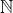, thenhas a finite infimum and it is an element of.

True

False

True

Explanation:

According to the Well-Ordered Principal this statement is true. The following proof illuminate its truth.

Suppose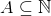is nonempty. From there, it is known that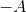is bounded above, by.

Therefore, by the Completeness Axiom the supremum ofexists.

Furthermore, if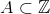has a supremum, then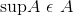, thus in this particular case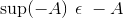.

Thus by the Reflection Principal,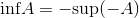exists and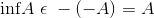.

Therefore proving the statement in question true.